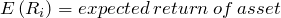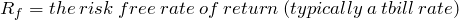## Tutorial: The Capital Asset Pricing Model

Apr 032011

Let’s begin with some Q&A:

Question: Why do some financial assets tend to earn higher returns than others?

Answer: Financial theory tells us that, on average, higher returns are earned by financial assets that have higher risk.

Question: Ok, so how do we measure risk?

Answer: One model used for measuring risk is the Capital Asset Pricing Model or CAPM.   In the CAPM, the risk that a security contributes to a diversified portfolio is measured by its beta (or), and the expected excess return of the security is proportional to beta.

The term “excess return” refers to the additional return that the security earns above the risk-free rate.  For U.S. investors, the t-bill rate is typically used as the risk-free rate.

The excess return earned by the market portfolio is also known as the “market risk premium”.  The risk premium for an individual security may be higher or lower than the market risk premium depending on the security’s beta.

If the beta is greater than one (the beta of the market portfolio is one by definition), then the expected return for the security is higher than the market average, and, if the beta is less than one, the expected return is lower.

In the CAPM, the total expected return of a security is given by this equation:Notice that only the excess return varies with beta, and the risk free rate is added to the security’s risk premium to get the total expected return.

The variables in this equation are:The “expected return” is the return that the investors expect to earn on average given the current price of the security.  Of course, the expected return is what should happen on average over the very long term, but in any given period the realized return may be very different than the expected return.

All else equal, the expected return is related to price.  If the price rises, and our expectations of the future cash flows from the security are unchanged, then our expected return falls since we are paying more for the same future future cash flows.  Similarly, if the price falls, and our expectations for future cash flows are unchanged, then the expected return will rise.